# Cyclotron

The cyclotron was one of the earliest types of particle accelerators, and is still used as the first stage of some large multi-stage particle accelerators. It makes use of the magnetic force on a moving charge to bend moving charges into a semicircular path between accelerations by an applied electric field. The applied electric field accelerates electrons between the "dees" of the magnetic field region. The field is reversed at the cyclotron frequency to accelerate the electrons back across the gap.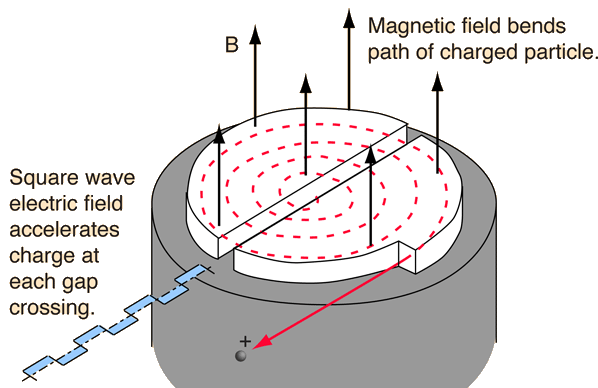When the cyclotron principle is used to accelerate electrons, it has been historically called a betatron. The cyclotron principle as applied to electrons is illustrated below.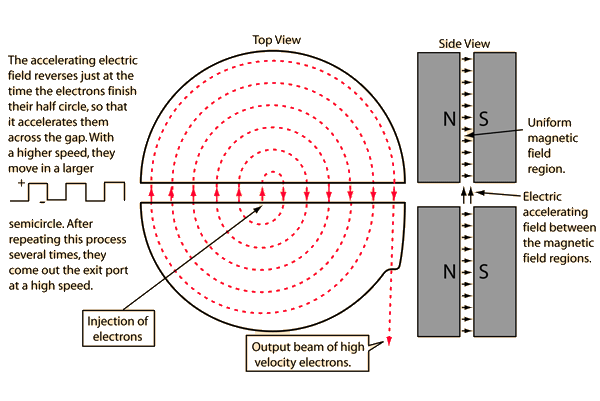Note: these illustrations are grossly simplified for demonstration of the cyclotron principle. In current practice sine waves are used for the acceleration and the "dees" are resonant cavities favoring one frequency. The magnetic fields are typically altered to keep the acceleration condition optimized, even when speeds become high enough that relativistic corrections are necessary.
 Magnetic interactions with charge Magnetic force applications
Index

Electromagnetic force

Magnetic field concepts

 HyperPhysics***** Electricity and Magnetism R Nave
Go Back

# Cyclotron Frequency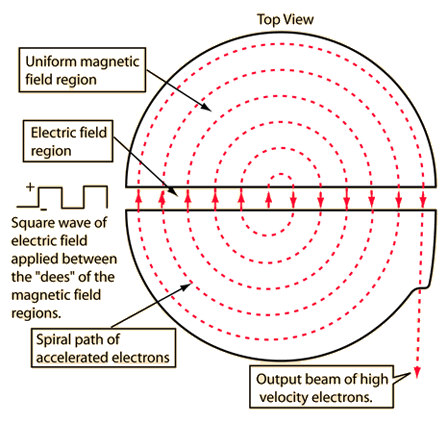A moving charge in a cyclotron will move in a circular path under the influence of a constant magnetic field. If the time to complete one orbit is calculated: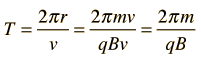it is found that the period is independent of the radius. Therefore if a square wave is applied at angular frequency qB/m, the charge will spiral outward, increasing in speed.

When a square wave of angular frequency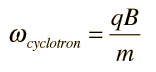is applied between the two sides of the magnetic poles, the charge will be boosted again at just the right time to accelerate it across the gap. Thus the constant cyclotron frequency can continue to accelerate the charge (so long as it is not relativistic).

Index

Electromagnetic force

Magnetic force

Magnetic field concepts

 HyperPhysics***** Electricity and Magnetism R Nave
Go Back